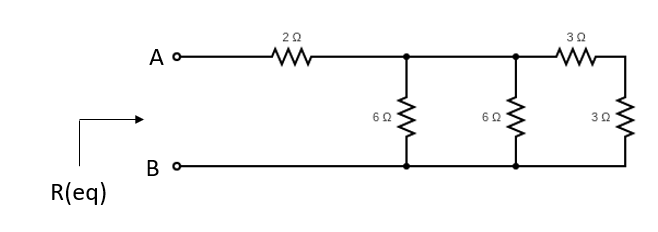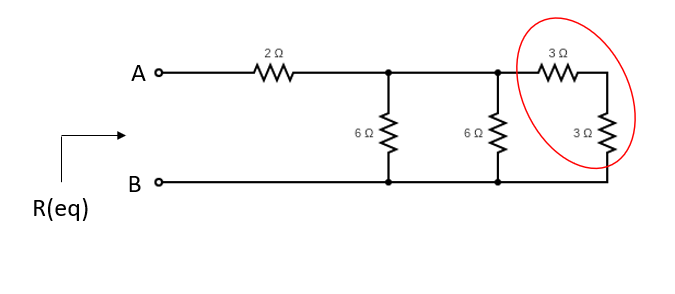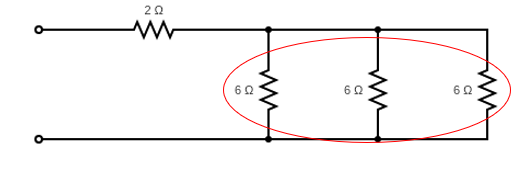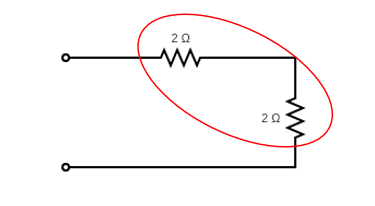## Resistance

In the below resistor circuit diagram, what is the equivalent resistance at terminals A-B?Hint
For resistors in Parallel:
$$\frac{1}{R_{T}}=\frac{1}{R_{1}}+\frac{1}{R_{2}}+\frac{1}{R_{3}}...+\frac{1}{R_{n}}$$$Hint 2 For resistors in Series: $$R_{T}=R_{1}+R_{2}+R_{3}...+R_{n}$$$
The problem statement is asking to find the total resistance in the circuit, which has certain sections both in series and in parallel.

The equivalent resistance for $$n$$ resistors in Parallel:
$$\frac{1}{R_{T}}=\frac{1}{R_{1}}+\frac{1}{R_{2}}+\frac{1}{R_{3}}...+\frac{1}{R_{n}}$$$The equivalent resistance for $$n$$ resistors in Series: $$R_{T}=R_{1}+R_{2}+R_{3}...+R_{n}$$$
Breaking down the diagram into smaller portions, let’s analyze the the highlighted section first.These two resistors are in series, so $$R_T=3+3=6\Omega$$ . The diagram can now be redrawn as:These three resistors are in parallel, so $$R_{T}=\frac{1}{\frac{1}{6}+\frac{1}{6}+\frac{1}{6}}=2\Omega$$ , so the redrawn diagram:Finally, the simplified diagram of the original problem are two resistors in series, meaning the equivalent resistance at Terminals A-B is $$R_T=2+2=4\:\Omega$$ .
4 Ω